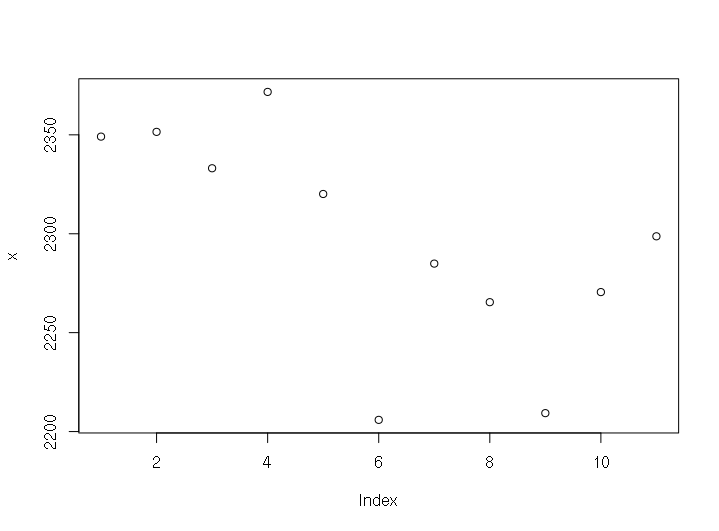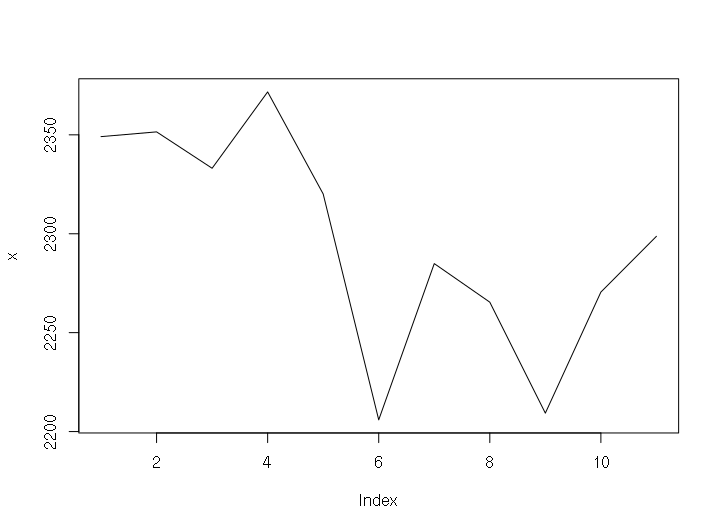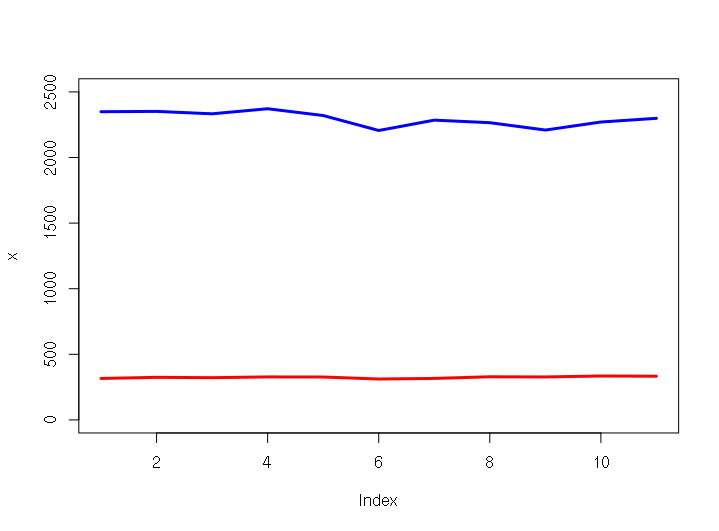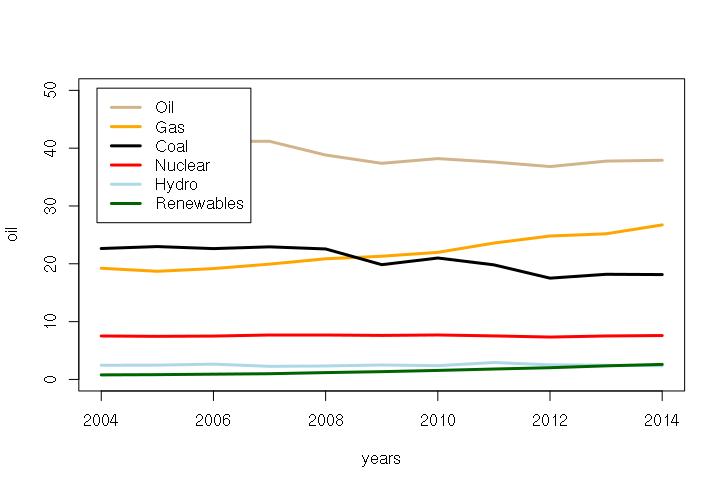# Introduction to RR is an open-source computer language and software environment for statistical analysis. R was first developed in 1993 by Ross Ihaka and Robert Gentleman at the University of Auckland, New Zealand. It based on the S statistical computing language that was originally developed at the legendary Bell Labs in 1976.

R is open-source, which means that the programming source code for the software is available for programmers to enhance and debug, as opposed to proprietary software like Micro\$oft Office or Adobe Photoshop, where the source code is developed by the in-house or contract programmers of a single company and guarded as a trade secret.

Open source programs are generally freely available to end users via the Internet and are supported by a global network of corporate and private programmers. This facilitates flexible enhancement and speedy bug repair, although it can also leave projects understaffed and orphaned.

Open source is about freedom in two senses of the word: free as in beer (no cost to acquire) and free as in speech (you can fix or modify the code if you have the skills). It is based on a collectivist ideology that technology advances best when we stand on each others shoulders rather than stand on each others toes.

R has a vast and growing collection of function libraries freely available from online repositories for performing a wide variety of data manipulations. There are numerous help forums online and you can get many (if not most) of your questions answered simply by doing a Google search.

## Reading This Tutorial

In the examples given in this tutorial, commands you type are displayed preceded by a > symbol that is a prompt from the program.

After you type a command you press the Enter or Return key to execute the command.

The examples show what R will display in response to your commands.

To get the full benefit of this tutorial, it is recommended that you actually type commands into R as you skim through the examples.

And working through the examples with a partner can be both enjoyable and mutually beneficial (peer instruction).

## Installing and Using R

R is installed on the lab computers. Use the 32-bit R rather than the 64-bit R (which sometimes has problems with external function libraries).

You may download R for PC or Mac on the Comprehensive R Archive Network (CRAN) home page if you wish to use it on your own machine.

When you start R, you are presented with a console. You interact with R almost exclusively with typed commands. While this is an unfamiliar way of working for many computer users and involves a learning curve, it is also a very fast and precise way of working with software once you gain some mastery of the basics.

This command-line mode of interaction is also helpful for working with large data sets. While Excel will open files with large numbers of rows or columns, it very slow and cumbersome to navigate through such data. R can load and store large data sets quickly, which makes R ideal for many "big data" analysis tasks.

The following is a brief overview of the R Graphical User Interface (GUI) on Windoze.

## Mathematical Expressions

At it's simplest, you can use R as a calculator and it will display the value.

```> 2+2
 4
```

R expressions are similar to formulas in Excel and use the same mathematical symbols or operators: + - * /. The carat operator (^) is used for exponents.

```> 2+2
 4
> 10 + 2
 12
> 10 - 2
 8
> 10 * 2
 20
> 10 / 2
 5
> 10^2
 100
```

## Variables

To make it possible to use the values of calculations in subsequent formulas, you can assign values to named variables. These are symbolic names you can use in later formulas to save the effort of repeating calculations, or simply to make formulas easier to read.

To display the value of a variable at the console, you simply type the name of the variable.

```> x = 10 * 2
> x
 20
> x + 15
 35
```

R has a "<-" operator that is also used to assign values to variables and you should know about this in case you look at code commonly shared on the web. This operator give R a distinctive look, but also makes it more difficult for casual R-users to read. Please use the equals sign rather than the <- operator in this class unless absolutely necessary.

```> y <- 12 + 16
> y
 28
> y = 12 + 16
> y
 28
```

Variable names must start with a letter and are case sensitive.

```> hello = 12
> Hello = 15
> hello
 12
> Hello
 15
```

You should always try to make your variable names meaningful so that you and other people can understand what your variables are. Rather than calling a variable containing a standard deviation "s" you might call it "stdev". The extra time spent typing now will save you confusion later.

Variables cannot contain spaces. However there are techniques for representing multi-word variable names that get around this issue:

• wordword
• word_word (underscore)
• word.word (period)
• wordWord (camelback capitalization)

One of the most powerful features of R is that variables can contain many different types of data. Variables can also contain text. Segments of text are called strings of characters. You assign text by enclosing your text in quotation marks.

```> x = "Hello"
> Hello = 12
> x
 "Hello"
> Hello
 12
```

## Vectors

In statistical calculations, we are commonly dealing with multiple numbers at the same time. One of the most powerful features of R is that it permits variables to contain multiple numbers at the same time. These collections of numbers are called vectors.

Vectors can be created by enclosing multiple numbers in the c() function (c is for combine).

```> x = c(1,3,5,7,10)
> x
  1  3  5  7 10
```

Note that variables by default are vectors in R. If you assign a single value to a variable, it is a vector of one element, which is why display of a single-valued variable is preceded by a "" indicating that it is the first (and only) value in the vector:

```> x=20
> x
 20
```

You can then perform multiple operations of the same kind on a vector with a single expression.

```> x
  1  3  5  7 10
> x + 2
  3  5  7  9 12
> x * 10
  10  30  50  70 100
> x - 5
 -4 -2  0  2  5
> x / 2
 0.5 1.5 2.5 3.5 5.0
> x ^ 2
   1   9  25  49 100
```

We commonly need to create vectors that contain a range of numbers. This can be done easily using the numeric range expression number:number.

```> x = 1:20
> x
  1  2  3  4  5  6  7  8  9 10 11 12 13 14 15 16 17 18 19 20
> x = 20:1
> x
 20 19 18 17 16 15 14 13 12 11 10  9  8  7  6  5  4  3  2  1
```

While range expressions can only be whole numbers (integers) and progress by +1 or -1, you can use mathematical operators to get other types of ranges.

```> x = 0:20
> x / 2
  0.0  0.5  1.0  1.5  2.0  2.5  3.0  3.5  4.0  4.5  5.0  5.5  6.0  6.5  7.0
  7.5  8.0  8.5  9.0  9.5 10.0
> (x + 10) * 10
 100 110 120 130 140 150 160 170 180 190 200 210 220 230 240 250 260 270 280
 290 300

```

## Built-In Functions

Functions in R are similar to functions in Excel. They have a name followed by a set of zero or more parameters in parentheses and separated by commas:

```name(parameter1, parameter2, ...)
```

R has dozens available with a default installation, and hundreds more that can be brought in from libraries that permit many different types of mathematical operations to be performed on many different types of data.

The basic descriptive functions are similar to those in Excel.

```> x = 1:20
> x
  1  2  3  4  5  6  7  8  9 10 11 12 13 14 15 16 17 18 19 20
> max(x)
 20
> min(x)
 1
> mean(x)
 10.5
> median(x)
 10.5
> sd(x) # standard deviation
 5.91608
```

Because R has functions for most common statistical operations, much of the work in using R simply involves finding the proper function to use and determining what parameters it needs.

## Custom Functions

R allows you to create custom functions that automate repetitive tasks and encapsulate complex mathematical operations.

In the simple example below, a new function mmtoe_to_quads is created that converts millions of tonnes of oil (MMTOE) to quadrillions of BTU using a simple conversion factor. The parameter to function() is used inside the function (defined by the braces), and the converted value is returned using the return() function.

```> mmtoe_to_quads = function(mmtoe) { return(mmtoe * 0.04) }
> mmtoe_to_quads(2000)
 80
```

The example above is very simple, and functions often consist of dozens of lines of code that would be enclosed in curly braces and terminate with a return() value.

## Plotting Vectors

The plot() function allows you to create a variety of different types of graphs. By default, when passed a single vector, the plot() function draws a point graph:

```> x = c(2349.1, 2351.5, 2333.1, 2371.7, 2320.1, 2205.9, 2284.9, 2265.4, 2209.3, 2270.5, 2298.7)
> plot(x)
```Example Default Point Plot

You can change that to a line graph by adding the parameter type="l":

```> x = c(2349.1, 2351.5, 2333.1, 2371.7, 2320.1, 2205.9, 2284.9, 2265.4, 2209.3, 2270.5, 2298.7)
> plot(x, type="l")
```Example Single-Line Plot

You can print multiple lines on a graph by calling plot() for the first series, then calling the lines() function for subsequent lines.

• The col parameter defines different colors for the different lines
• The lwd parameter increases the line width so they are more visible
• The ylim parameter in the plot defines the limits (range) for the Y-axis, so the smaller values in the added lines are visible
```> x = c(2349.1, 2351.5, 2333.1, 2371.7, 2320.1, 2205.9, 2284.9, 2265.4, 2209.3, 2270.5, 2298.7)
> plot(x, type="l", col="blue", lwd=3, ylim=c(0,2500))
>
> y = c(315.6, 324.2, 321.6, 327.4, 326.7, 311.3, 315.9, 328.5, 327.2, 334.3, 332.7)
> lines(y, col="red", lwd=3)
```Example Multiple-Line Plot

## Scripts

Another powerful advantage of a command-line console is that you can type commands into script files and run them. This makes it possible to easily repeat complex sequences of operations. And if you find an error in your script, you can fix it and rerun the script without the labor of having to repeat long sequences of button clicks that you would need with a graphical user interface like ArcMap.

R on the PC contains a simple script editor. To create a script on the PC, select File -> New Script. The video above shows how to run a script from

On the Mac you will need a plain text editor like TextWrangler.

You can also use a text editor like notepad or notepad++ on the PC, or Micro\$soft Word on any platform, although you need to make sure that the file is saved as a plain text file, since R cannot understand the complex formatting of .docx files.

If you are using a version of R that does not have a GUI, you can execute scripts from the console using the source() function with the file name of the script:

```source('script.r')
```

By default, the source() command does not print the commands as they are executed. You can enable echoing of commands with the echo parameter:

```source('script.r', echo=T)
```

### Comments

Comments in scripts are lines that the program ignores. These lines are used for documenting the authorship of scripts and for adding comments that explain what is going on when you have complex sequences of expressions and function calls.

Comments start with a pound sign (#) and tell the R interpreter to ignore everything that follows on that line.

```# Name of script (date)
# This is a comment that explains what the line after it does
x = 2 + 2
```

### Output From Scripts

If you

You may note that the mean() function does not print out anything when run in a script. You need to use a function like cat() to have scripts display the contents of variables. cat() takes an unlimited number of parameters, concatenates them, and displays them on the console. The last parameter should be a newline or "\n" to start a new line at the end of what is printed. Type:

```cat("Mean temperature:", mean(weather\$Mean.TemperatureF), "\n")
```

Some functions display their output as a table and a function like cat() will only display a list of values. For example, the quantile() function displays a table of quantile values. To get that table to print from a script, you need to use the print() function. Type:

```print(quantile(weather\$Mean.TemperatureF))
```

If you want to display multiple plot() from a script, you need to add a dev.new() function to open a new window. Otherwise, each subsequent plot erases the previous one. Putting all this together, modify your script as follows and run it from the console.

### Example Script: The BP Statistical Review

Each year, the multinational energy company BP releases The BP Statistical Review of World Energy with a vast amount of information on energy resources, production and consumption in countries around the world.

One challenge with BP data is that, since they are primarily an oil company, they report data in terms of amounts of oil, which is different from the quadrillions of British thermal units (quads) used by the US Department of Energy for reporting national statistics. However, there are conversion factors we can use in an R script to perform conversions and graph data for analysis of fuel mix over the period 2004 to 2014 for the USA:

```# US Energy Statistics - BP Statistical Review
# Michael Minn - 12 February 2017

years = 2004:2014

# Oil is in thousands of barrels per day.
# 1000 barrels of oil = 0.000005456 quads

oil = c(20732, 20802, 20687, 20680, 19490, 18771, 19180, 18882, 18490, 18961, 19035)
oil = oil * 365 * 0.000005456

# All other energy sources are in millions of tonnes of oil equivalent (MTOE)
# Each million tonnes of oil is approximately 0.04 quadrillion BTU
mtoe_to_quads = 0.04

gas = c(480.7, 467.6, 479.3, 498.6, 521.7, 532.7, 549.5, 589.8, 620.2, 629.8, 668.2)
gas = gas * mtoe_to_quads

coal = c(566.1, 574.5, 565.7, 573.3, 564.2, 496.2, 525.0, 495.4, 437.9, 454.6, 453.4)
coal = coal * mtoe_to_quads

nuclear = c(187.8, 186.3, 187.5, 192.1, 192.0, 190.3, 192.2, 188.2, 183.2, 187.9, 189.8)
nuclear = nuclear * mtoe_to_quads

hydro = c(61.3, 61.8, 66.1, 56.6, 58.2, 62.5, 59.5, 73.0, 63.1, 61.4, 59.1)
hydro = hydro * mtoe_to_quads

renewables = c(19.6, 20.6, 22.7, 24.7, 29.5, 33.6, 38.9, 45.0, 50.6, 58.7, 65.0)
renewables = renewables * mtoe_to_quads

# Graph in different colors
# The lwd parameter sets line width
# The col parameter sets line color
# The ylim parameter sets the range of the Y-axis

plot(x=years, y=oil, col="tan", type="l", lwd=3, ylim=c(0, 50))
lines(x=years, y=gas, col="orange", lwd=3)
lines(x=years, y=coal, col="black", lwd=3)
lines(x=years, y=nuclear, col="red", lwd=3)
lines(x=years, y=hydro, col="lightblue", lwd=3)
lines(x=years, y=renewables, col="darkgreen", lwd=3)

legend(x="topleft", legend=c("Oil","Gas","Coal","Nuclear","Hydro","Renewables"),
col=c("tan","orange","black","red","lightblue","darkgreen"),
inset=0.03, lty=1, lwd=3, bg="white")
```US Energy Source Mix 2004-2014 (Source: BP)

## Getting Help

From within the R console, you can get documentation for most functions by typing a question mark followed by the name of the function. Type:

```?mean
```

If you have only a vague idea of what you want, you can type a double question mark and a keyword. Type:

```??normal
```

If you have any more complex questions about the language syntax or a cryptic error message issued by the program, there is a vast amount of R information on a variety of Internet websites. The Google is your tech support for R.